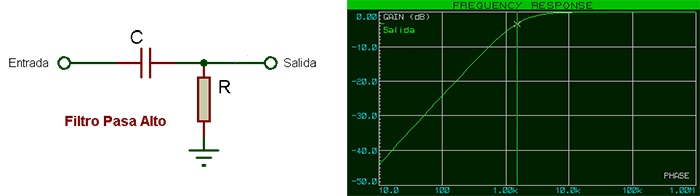# Gzalo

ES · EN

## RC filter calculator

Given the value of the resistor and capacitor, this page calculates the cutoff frequency of a series RC passive filter

R: Ohms

F:The curve rate is 20 decibels per decade. At the cutoff frequency, the output voltage is 3 decibels (`0.707 times`) below the input voltage.

If the required capacitance is too big, and the resistance value can’t be increased, it might be easier to implement the filter actively, using an opamp. This site provides a calculator for that purpose.

If the load impedance is around the filter impedances, the cutoff filter will be different from the one wanted. In that case, a simple analog buffer may be added, for instance by using an opamp as a voltage follower, in such a way that the load doesn’t affect the filter itself.